Vector Scalar MultiplicationThe scalar multiplication of vectors is also a relatively simple matter for vector math instructions to handle, just like the scalar addition and subtraction of vectors. The trick is to replicate the scalar so it appears like a second vector.

Pseudo Vec

Single-Precision Vector Float Multiplication with Scalar

This function multiplies a scalar with each element of a vector. A scalar has multiple uses but the primary is in the use of "scaling" a vector. A scalar of one would result in the same size . Two would double the length of the vector, etc.

Listing 13-11: ...\chap13\vmd3d\Vmd3D.cppvoid vmp_VecScale(vmp3DVector * const pvD,             const vmp3DVector * const pvA,             float fScalar) {   pvD>x = pvA>x * fScalar;   pvD>y = pvA>y * fScalar;   pvD>z = pvA>z * fScalar; }Single-Precision Quad Vector Float Multiplication with Scalar

Listing 13-12: ...\chap13\qvmd3d\QVmd3D.cppvoid vmp_QVecScale(vmp3DQVector * const pvD,            const vmp3DQVector * const pvA,            float fScalar) {   pvD>x = pvA>x * fScalar;   pvD>y = pvA>y * fScalar;   pvD>z = pvA>z * fScalar;   pvD>w = pvA>w * fScalar; }Pseudo Vec (x86)

mov   eax,vA         ; Vector A mov   edx,vD         ; Vector destination

vmp_VecScale (3DNow!)

The 32-bit scalar is unpacked into a pair and then treated similar to the vector multiplication of two vectors.

Listing 13-13: \chap13\vmd3d\Vmd3DX86M.asmmovd       mm0,fScalar                       ; fScalar {0 s} punpckldq  mm0,mm0                           ; {s s} movq       mm1,[eax]                         ; vA.xy {Ay Ax} movd       mm2,(vmp3DVector PTR [eax]).z     ; {0   Az} pfmul      mm1,mm0                           ; {Ays Axs} pfmul      mm2,mm0                           ; {0s   Azs} movq       [edx],mm1                         ; {Ays Axs} movd       (vmp3DVector PTR [edx]).z,mm2     ; {       Azs}vmp_VecScale (SSE) Aligned

The SSE version of the code is changed from a 64-bit load to a 128-bit load, but the principles remain the same.

Listing 13-14: \chap13\vmd3d\Vmd3DX86M.asmpxor    xmm1,xmm1                      ; {0 0 0 0} movss   xmm1,fScalar                   ; {0 0 0 s}  movaps  xmm2,[edx]                     ; {Dw # # #}  movaps  xmm0,[eax]                     ; vA.xyz# {# Az Ay Ax} shufps  xmm1,xmm1,11000000b            ; 3 0 0 0 {0   s   s   s} andps   xmm2,OWORD PTR himsk32         ; {Dw 0   0   0}     mulps   xmm0,xmm1                      ; {#   Azs Ays Axs} andps   xmm0,OWORD PTR lomsk96         ; {0   Azs Ays Axs} orps    xmm0,xmm2                      ; {Dw Azs Ays Axs}  movaps  [edx],xmm0                     ; {Dw Azs Ays Axs}vmp_QVecScale (SSE) Aligned

Listing 13-15: \chap13\qvmd3d\QVmd3DX86M.asmmovss   xmm1,fScalar               ; {0 0 0 s} movaps  xmm0,[eax]                 ; vA.xyzw {Aw Az Ay Ax} shufps  xmm1,xmm1,00000000b        ; 0 0 0 0 {s s s s} mulps   xmm0,xmm1                  ; {Aws Azs Ays Axs} movaps  [edx],xmm0                 ; {Aws Azs Ays Axs}I-VU-Q

What is the difference between a dot product and a cross product and what are their equations?

Graphics 101 Dot Product

A dot product, also known as an inner product , of two vectors is the summation of the results of the product for each of their {XYZ} elements, thus resulting in a scalar. Not to oversimplify it, but this scalar is equal to 0 if the angle made up by the two vectors are perpendicular (=90), positive if the angle is acute (<90), and negative if the angle is obtuse (>90).

v ={v 1 , v 2 , v 3 } and w = {w 1 , w 2 , w 3 }

These are vectors that produce a scalar defined by v w when their products are combined. The dot product is represented by the following equation:

v w = v 1 w 1 + v 2 w 2 + v 3 w 3

The equation resolves to the following simplified form:

D = A x B x + A y B y + A z B z

D = Ax*Bx + Ay*By + Az*Bz;

Pseudo Vec

So as we have learned, we first write it in a high-level language before writing it in assembly code.

Single-Precision Dot Product

Listing 13-16: ...\chap13\vmd3d\Vmd3D.cppvoid vmp_DotProduct(float * const pfD,         const vmp3DVector * const pvA,         const vmp3DVector * const pvB) {   *pfD = pvA>x * pvB>x        + pvA>y * pvB>y        + pvA>z * pvB>z; }This is one of my favorite equations because it does not slice, dice, or chop, but it culls, it illuminizes, it simplifies , it cosineizes (not a real word, but you know what I mean). It is the Sledge-O-Matic!!! Well, not quite comedian Gallagher's watermelon disintegration kitchen utensil, but it does do many things and so it is just as useful.

From Figure 13-1 you will note that if the resulting scalar value is positive (+), the vectors are pointing in the same general direction. If zero (0), they are perpendicular to each other, and if negative (), they are pointed in opposite directions.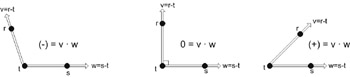Figure 13-1: Dot product (inner product). A positive number is an acute angle, zero is perpendicular, and negative is an obtuse angle.

Before explaining further it should be pointed out that to keep 3D graphic algorithms as simple as possible the three vertices for each polygon should all be ordered in the same direction. For example, by using the left-hand rule and keeping all the vertices of a visible face in a clockwise direction, such as in Figure 13-2, back face culling will result. If all visible face surfaces use this same orientation, then if the vertices occur in a counterclockwise direction they are back faced and thus pointing away and need not be drawn, saving render time.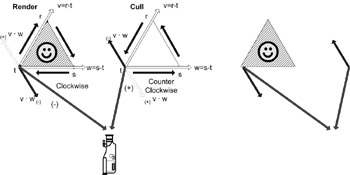Figure 13-2: Face culling mechanism where if the angle between the camera and the perpendicular to the face plane is obtuse, then the face is pointed away from the camera and can be culled.

Contrarily, if polygons are arranged in a counterclockwise orientation, then the inverse occurs where a positive value is drawn and a negative value is culled. Keep in mind, however, that most software algorithms keep things in a clockwise orientation.

By calculating the dot product of the normal vector of the polygon with a vector between one of the polygon's vertices and the camera, it can be determined if the polygon is back facing and needs to be culled. A resulting positive value indicates that the face is pointed away, hence back facing and can be culled and not rendered. A negative value indicates a face oriented toward the camera and thus visible.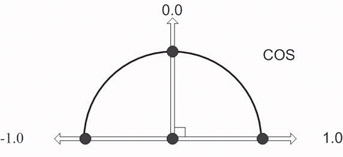Figure 13-3: This shows the cosine of two intersecting lines.

Another use for the dot product equation is that it is also the cosine of the angle. The cosine is returned by dividing the dot product by the product of the magnitudes of the two vectors. Note that v and w are vectors and that v and w are their magnitudes.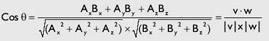And using standard trigonometric formulas, such as:

1 = Cos 2 + Sin 2

sine and other trigonometric results can be calculated.

So the good stuff is yet to come!

Pseudo Vec (x86)

vmp_DotProduct (3DNow!)

The 3DNow! instruction set uses the 64-bit MMX registers, but 64-bit memory alignment cannot be guaranteed . In this case, it is typically better to handle memory access as individual 32-bit floats then to unpack into 64-bit pairs, process, then save individually as 32 bit. The PFACC instruction is unique as it allows the hi/lo 32 bits to be summed with each other, within each of the vectors.

Listing 13-17: \chap13\vmd3d\Vmd3DX86M.asmmov     ebx,vB                        ; Vector B mov     eax,vA                        ; Vector A mov     edx,vD                        ; Vector destination     movd    mm0,(vmp3DVector PTR [ebx]).z ; {0 Bz} movd    mm1,(vmp3DVector PTR [eax]).z ; {0 Az} movq    mm2,[ebx]                     ; {By Bx} movq    mm3,[eax]                     ; {Ay Ax} pfmul   mm0,mm1                       ; {00 BzAz} pfmul   mm2,mm3                       ; {ByAy BxAx}  pfacc  mm2,mm2                       ; {ByAy+BxAx ByAy+BxAx} pfadd   mm0,mm2                       ; {ByAy+BxAx+0 ByAy+BxAx+BzAz} movd    [edx],mm0                     ; Save {ByAy+BxAx+BzAz}vmp_DotProduct (SSE) Aligned

The SSE instruction loads the 96-bit vector value using a 128-bit XMM register. The operation entails the multiplication of the {XYZ} pairs from both vectors. The data is swizzled to allow scalar additions, and then the 32-bit single-precision float scalar result is written to memory.

Listing 13-18: \chap13\vmd3d\Vmd3DX86M.asmmovaps  xmm1,[ebx]                  ; vB.xyz# {# Bz By Bx}  movaps  xmm0,[eax]                  ; vA.xyz# {# Az Ay Ax} mulps    xmm0,xmm1                   ; {A#B# AzBz AyBy AxBx} movaps   xmm1,xmm0 movaps   xmm2,xmm0 unpckhps xmm0,xmm0                   ; {A#B# A#B# AzBz AzBz} shufps   xmm1,xmm1,11100001b         ; {A#B# AzBz AxBx AyBy} addss    xmm2,xmm0                   ; {A#B# AzBz AxBx AzBz+AxBx} addss    xmm2,xmm1                   ; {A#B# AzBz AxBx AzBz+AxBx+AyBy} movss    [edx],xmm2                  ; Save {AzBz+AxBx+AyBy}Graphics 101 Cross Product

A cross product, also known as the outer product , of two vectors is a third vector perpendicular to the plane of the two original vectors. The two vectors define two sides of a polygon face and their cross product points away from that face.Figure 13-4: Cross product (outer product). The perpendicular to the two vectors v and w.

v ={v 1 , v 2 , v 3 } and w = {w 1 , w 2 , w 3 } are vectors of a plane denoted by matrix R 3 . The cross product is represented by the following equation:

The standard basis vectors are i =(1,0,0) j =(0,1,0) k =(0,0,1).

v w = (v 2 w 3 v 3 w 2 ) i (v 1 w 3 v 3 w 1 ) j + (v 1 w 2 v 2 w 1 ) kThe equation resolves to the following simplified form:

D x = A y B z A z B y     Dx = Ay*Bz Az*By;

D y = A z B x A x B z     Dy = Az*Bx Ax*Bz;

D z = A x B y A y B x     Dz = Ax*By Ay*Bx;

Note the following simple vector structure is actually 12 bytes, which will pose a data alignment problem for SIMD operations.

One method is to use individual single-precision floating-point calculations, of which you may already be familiar. With this in mind, examine the following simple C language function to implement it. Note the use of the temporary floats x , y to prevent the resulting solutions of each field {x,y,z} from affecting either source pvA or pvB in the case where the destination pvD is also a source.

Listing 13-19: ...\chap13\vmd3d\Vmd3D.cppvoid vmp_CrossProduct(vmp3DVector* const pvD,    const vmp3DVector* pvA, const vmp3DVector* pvB) {   float x, y;         x = pvA>y * pvB>z  pvA>z * pvB>y;         y = pvA>z * pvB>x  pvA>x * pvB>z;   pvD>z = pvA>x * pvB>y  pvA>y * pvB>x;   pvD>x = x;   pvD>y = y; }vmp_CrossProduct (3DNow!)

The 3DNow! instruction set uses the 64-bit MMX registers, but 64-bit memory alignment cannot be guaranteed. In this case it is typically better to handle memory access as individual 32-bit floats than to unpack into 64-bit pairs, process, then save individually as 32 bit. This example is kind of big so there are extra blank lines to help separate the various logic stages and it is not optimized to make it more readable.

Listing 13-20: \chap13\vmd3d\Vmd3DX86M.asmmov    ebx,vB                          ; Vector B mov    eax,vA                          ; Vector A mov    edx,vD                          ; Vector destination     movd mm0,(vmp3DVector PTR [ebx]).x   ; vB.x {0 Bx} movd mm1,(vmp3DVector PTR [ebx]).y   ; vB.y {0 By} movd mm2,(vmp3DVector PTR [ebx]).z   ; vB.z {0 Bz} movd mm3,(vmp3DVector PTR [eax]).x   ; vA.x {0 Ax} movd mm4,(vmp3DVector PTR [eax]).y   ; vA.y {0 Ay} movd mm5,(vmp3DVector PTR [eax]).z   ; vA.z {0 Az}     pfmul mm4,mm0                        ; vB.xy {0 AyBx} punpckldq mm0,mm1                    ; {By Bx}     movd mm1,(vmp3DVector PTR [eax]).y ; vA.y {Ay} movd mm6,(vmp3DVector PTR [ebx]).y ; vB.y {By}     punpckldq mm2,mm2                  ; {Bz Bz} punpckldq mm3,mm1                  ; {Ay Ax} punpckldq mm5,mm5                  ; {Az Az}     pfmul mm2,mm3                      ; vA.xy {BzAy BzAx} pfmul mm5,mm0                      ; vB.xy {AzBy AzBx} pfmul mm6,mm3                      ; vA.xy {0Ay ByAx}     movq   mm7,mm2                     ; {BzAy BzAx} pfsub mm2,mm5                      ; {BzAyAzBy BzAxAzBx}     psrlq mm2,32                       ; x@ {0 BzAyAzBy} pfsub mm5,mm7                      ; y@ {AzByBzAy AzBxBzAx} pfsub mm6,mm4                      ; z@ {00 ByAxAyBx}     movd (vmp3DVector PTR [edx]).x,mm2 ; x=AyBzAzBy movd (vmp3DVector PTR [edx]).y,mm5 ; y=AzBxAxBz movd (vmp3DVector PTR [edx]).z,mm6 ; z=AxByAyBxIf you examine it closely you will notice the operations performed within each block and how they correlate to the generic C code that was provided.

vmp_CrossProduct (SSE) Aligned

The SSE instruction set uses the 128-bit XMM registers with MOVUPS instead of MOVAPS for unaligned memory. This function has also been unoptimized so as to make it more readable.

Listing 13-21: \chap13\vmd3d\Vmd3DX86M.asmmovaps  xmm1,[ebx]                     ; vB.xyz# {# Bz By Bx}  movaps  xmm0,[eax]                     ; vA.xyz# {# Az Ay Ax} ;  Crop the 4  th  (w) field andps  xmm1,OWORD PTR lomsk96         ; {0 Bz By Bx} andps  xmm0,OWORD PTR lomsk96         ; {0 Az Ay Ax}     movaps xmm5,xmm1 movaps xmm6,xmm0     shufps xmm1,xmm1,11010010b            ; 3 1 0 2 {0 By Bx Bz} shufps xmm0,xmm0,11001001b            ; 3 0 2 1 {0 Ax Az Ay} shufps xmm6,xmm6,11010010b            ; 3 1 0 2 {0 Ay Ax Az} shufps xmm5,xmm5,11001001b            ; 3 0 2 1 {0 Bx Bz By}  movaps  xmm2,[edx]                     ; Get destination {Dw # # #} mulps  xmm1,xmm0 mulps  xmm5,xmm6 andps  xmm2,OWORD PTR himsk32         ; {Dw 0 0 0} subps  xmm1,xmm5                      ; { 0 z y x} orps   xmm1,xmm2                      ; [Dw z y x}  movups  [edx],xmm1                     ; vD.wxyz {Dw z y x}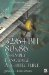32/64-Bit 80x86 Assembly Language Architecture
ISBN: 1598220020
EAN: 2147483647
Year: 2003
Pages: 191

Similar book on Amazon